Next: Differential Equations Up: Random WalksMarkov Chains Previous: NonUniform Distributions

# Monte Carlo Integration

We will first look at using Monte Carlo to evaluate definite integrals. There are two major Monte Carlo techniques for evaluating such integrals. The first method is based upon an idea similar to the rejection method of generating random variables for arbitrary distribution functions. Suppose we wish to evaluate the integral,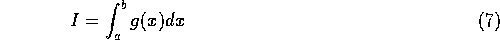If we put a bounding box around the function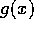, then the integral ofcan be understood to be the fraction of the bounding box that is also within. So if we choose a point at random uniformly within the bounding box, the probability that the point is withinis given by the fraction of the area thatoccupies. The integration scheme is then to take a large number of random points with the box and count the number that are withinto get the area,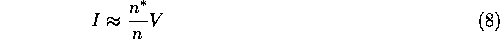where,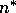is the number of points within, n is the total number of points generated, and V is the volume of the bounding box.

This method is very inefficient, many points are required to make (8) converge towards (7) with any degree of precision.

A more efficient approach is to note that we can write (7) as,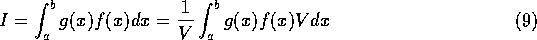if we define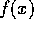as,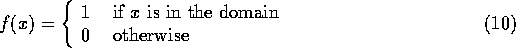(again V is the volume of the domain). (9) can be interpreted as the expectation of the function,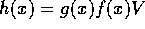, for the random variable x which is uniformly distributed within the domain. This then gives an approximate proceedure,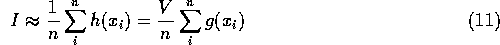Estimates based upon (11) converge much more quickly than those based upon using equation (8).

If pseudo-random numbers are used for the Monte Carlo evaluation of integrals then, because of the clumps and voids in any given sample, there will be regions of the integral that are under represented as well as overrepresented. In the long run it is not a problem since we know that the numbers represent a uniform distribution well. But ``the long run'' means using lots of iterations.

Probably the most effective way to speed up the convergence of Monte Carlo integration is to use quasi-random numbers instead of pseudo-random numbers for choosing the points. In general this change will cause the integration estimate to converge towards the actual solution like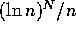(where N is the number of dimensions in the integral) instead of the usual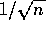. This improved convergence is considerably better, almost as fast as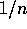.

Consider for example, the evaluation of the 2 dimensional integral,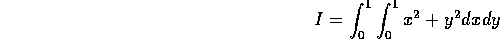A test of 5000 iterations using quasi-random numbers gives a value of 0.6664 (the exact value is 2/3). The same calculation using the same number of iterations with pseudo-random numbers, gives an estimate of 0.6632.Next: Differential Equations Up: Random WalksMarkov Chains Previous: NonUniform Distributions

Skip Carter
Tue Jan 9 12:38:54 PST 1996